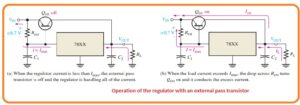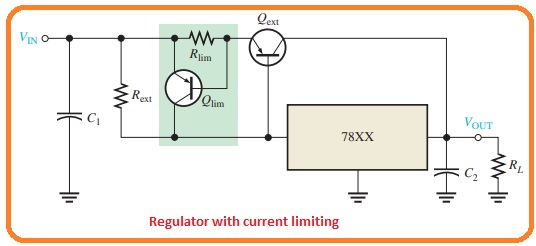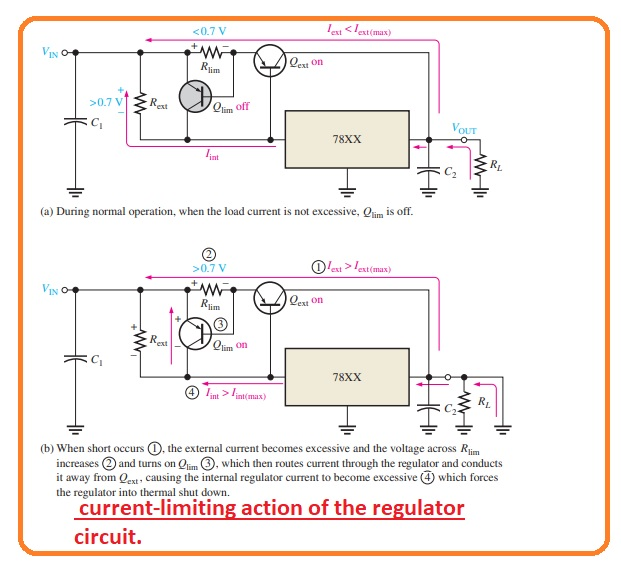Hello, readers welcome to another interesting post in this post we will have a detailed look at Applications of Integrated Circuit voltage regulators. In the last tutorial, we have discussed different types of integrated voltage regulators.

In this post numerous techniques are used to modify these circuits to enhance their operations. So let’s get started with Applications of Integrated Circuit voltage regulators.

External Pass Transistor

• The integrated circuit voltage regulator is able to offer a specific quantity of current at the load.
• For instance, the 78XX series regulators can hold a peak output current value of 1.3 amperes.
• If the load current larger than the extreme permittable value there will be thermal overloading and the regulator will be off.
• The thermal overloading states indicates that there is large power dissipation in the module.
• If there is such an application needed larger than the extreme current which regulates can provide an exterior pass transistor Qext can use.
• The below figure defines the 3 terminal regulators with the exterior pass transistor for holding current in a large amount of output current ability of the normal regulator.• The parameter of exterior current detecting resistance Rext finds the value of current at Qext start to operate since it adjusts the base to emitter voltage of the transistor.
• As the current is less than the values adjust with the Rext the transistor Qext is in off state and the regulator work in the normal way as can be seen in below figure.• f It is since the voltage loss about the Rext is less than the value of 0.7volts base to emitter voltage need to on the Qext.
• The Rext finds with the given expression here Imax is the larges current which the voltage regulator is to hold innerly.

Rext= 0.7 V/Imax

• When eh value of current is enough to generate 0.7 volts loss about Rext the outer pass transistor Qext gets s on and operates any current large of Imax can be seen in the above figure denoted as b.
• The Qext will operate larger of less relying on the load needs.
• For instance, if the load current is three amperes and Imax was restricted to one ampere the exterior pass transistor will operate two amperes that is larger than the inner voltage regulator current Imax.

Current Limiting

• The disadvantage of the circuit shown in first figure is that the outer transistor in not save from large value current like as current generated due to short circuit.
• The extra current limiting circuitry can be linked as shown in below figure.• To provide protection to Qext from large value current and getting burned out.
• There is a method is discusses the current limiting circuitry operates.
• The current detecting resistance Rlim adjusts the value of VBE fo transistor Qlim.
• The base to emitter voltage of Qext is no find with the Vrext-VRlim since they have reverse polarities.
• For normal working, the loss about the Rext should be enough to control the reverse loss about the Rlim.
• If the current through Qext larger than the specific extreme Iext(max) since due to short circuit output or errored load the voltage about Rlimm becomes 0.7 volts and on the Qlim.
• Qlim now operates current by the regulator and away from Qext causes the thermal overload to exist and off the regulator.
• Note that the IC regulator is innerly saved from thermal overloading as it is part of the structure.
• This process is explained in the below figure.
• In the figure denoted as a the circuitry is working generally with the Qext conducting less than the extreme current that it can bear by the Qlim in off.
• In figure denoted as b it occurs that there is short circuit about the load.
• The current about the Qext rises abruptly and cuases the voltage loss about Rlim to rises that turn Qlim on.
• The current now changes direction from the regulator that causes it to off due to thermal overloading.#### Current Regulator

• The 3 terminal regulators can be operated as a current source when application need which constant current can be send to the variable load.
• The circuit of this arrangement is shown in the below figure.• The regulator offers a fixed constant voltage among the ground terminal and output points.
• It finds the constant current to the load.
• IL= VOUT/R1 +IG

That is a detailed post about Applications of Integrated Circuit voltage regulators if you have any further query ask in the comments. Thanks for reading. Have a nice day.